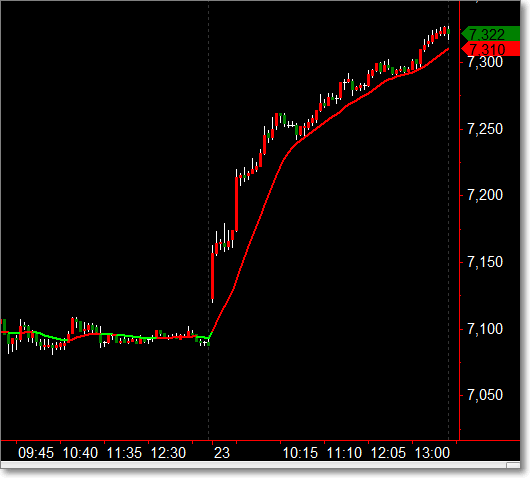2012年11月24日 星期六

多空線多空線，這個名稱我接觸到的開始已經忘記了，在我做過的 代工Case 中，有好些次有使用到這個所謂的多空線。而這個所謂的多空線在網路上也很容易查到計算公式，也就是它是一條公開的均線，基本上只是一條經過加權計算的均線，並不是具有自適化功能的均線。至於，在受託的 Case 中，大致上是怎樣使用這條多空線的，那就恕難透露了。

```value1=(3*C+O+H+L)/6;
value2=20*value1+19*value1+18*value1+...+1*value1;
value3=value2/210;```畢竟多空線只是一種加權計算的方式，要計算幾根K棒的價格，總是可以參數化的吧，如果現在想看20根，就用上面那個方式去 coding ，那要看 30根、60根呢？

``````input:len(Numeric);
var:step(0);

value1=(3*C+L+O+H)/6;
value2=0;
value3=0;

for step= 0 to len-1 begin
value2=value2+ (len-step)*value1[step];
value3=value3+(step+1);
end;

_BullBearMA= value2/value3;
``````

``````input:Len(20);

if _BullBearMA(len) > _BullBearMA(len) then
value1=RGB(255,0,0);
if _BullBearMA(len) < _BullBearMA(len) then
value1=RGB(0,255,0);
if _BullBearMA(len) = _BullBearMA(len) then
value1=RGB(0,0,0);

plot1( _BullBearMA(len),"theMA", value1);
````````````input:Len(20);

if _BullBearMA(len) > _BullBearMA(len) then
value1=RGB(255,0,0);
if _BullBearMA(len) < _BullBearMA(len) then
value1=RGB(0,255,0);
if _BullBearMA(len) = _BullBearMA(len) then
value1=RGB(0,0,0);

plot1(Average( _BullBearMA(len), len),"theMA",value1);
``````